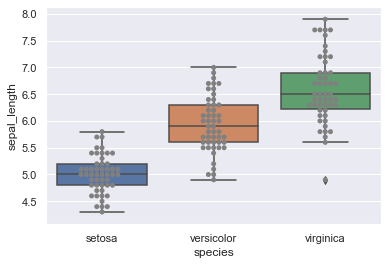Seaborn boxplot() function does not include any argument to display points directly. To do so, we use a matplotlib.axes object in order to successively plot a seaborn boxplot() and a seaborn swarmplot(). The latter enables us to add points to the figure.
Overall, such a figure is quite similar to a violinplots in terms of information.

``````# libraries & dataset
import seaborn as sns
import matplotlib.pyplot as plt
sns.set(style="darkgrid")

# Usual boxplot
ax = sns.boxplot(x='species', y='sepal_length', data=df)

# Add jitter with the swarmplot function
ax = sns.swarmplot(x='species', y='sepal_length', data=df, color="grey")
plt.show()``````## Contact & Edit

👋 This document is a work by Yan Holtz. You can contribute on github, send me a feedback on twitter or subscribe to the newsletter to know when new examples are published! 🔥